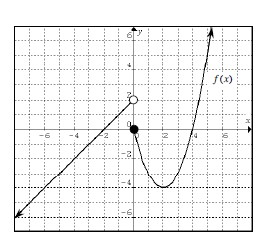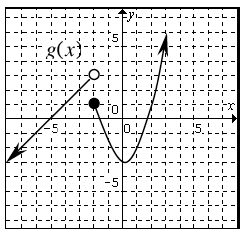### Home > PC > Chapter 3 > Lesson 3.2.1 > Problem3-65

3-65.

Given the function $f\left(x\right)$ below, answer the following questions.1. Find the piecewise defined function that will generate the graph of $f\left(x\right)$.

Use the vertex $\left(2,−4\right)$ to help find the equation of the parabola.

$f(x) = \begin{cases}x +2\text{ for } x < 0\\\:\:\:???\:\text{for}\:\textit{x} \geq 0\end{cases}$

2. Sketch the graph of $g\left(x\right) = f\left(x + 2\right) + 1$.